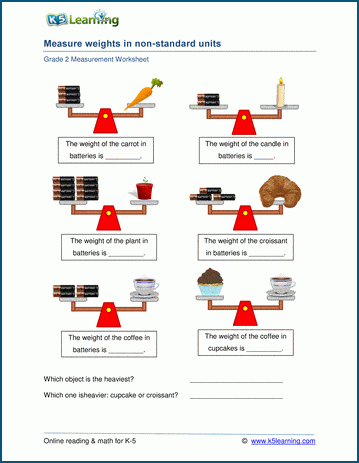# Measurement Worksheets

## Measurement worksheets for kindergarten through grade 6

Our measurement worksheets begin with the concepts of size comparison (e.g. "longer vs shorter") and measuring in non-standard units ("The pencil is 3 erasers long") and progresses to measuring length, weight, capacity and temperature in customary and metric units.

Kindergarten measurement worksheets

Kindergarten size comparison worksheets

Topics include:

## Kindergarten measurement worksheets

• Measuring lengths of an object pictured beside a scale (no units)
• Measuring lengths with a cut-out scale (no units)
• Measure and color lengths (no units)
• Measure lengths with a ruler (inches or centimeters)

## Kindergarten size comparison worksheets

• Bigger vs smaller
• Biggest and smallest
• Taller vs shorter
• Tallest and shortest
• Longer vs shorter
• Longest and shortest
• Same size vs different size

• Which object is shorter / longer?
• Ordering 3 objects by length
• Measuring length in non-standard units
• Measuring length in inches, centimeters
• Measuring lengths with a ruler
• Comparing lengths by measuring
• Which object is heavier / lighter?
• Which object holds more / less (capacity)?
• Combining and comparing lengths word problems (in, cm)

• Using benchmarks to measure lengths
• Measuring in non-standard units
• Measuring in inches and centimeters
• Estimating and measuring
• Differences in length
• Units of length (customary)
• Units of length (metric)
• Comparing weights using a balance scale
• Weights in non-standard units
• Weights in ounces / pounds / kilograms
• Capacity - more or less than 1 gallon
• Capacity - more or less than 1 liter
• Temperature - reading a thermometer

• Units of length (customary - inches, feet, yards, miles)
• Units of length (metric - cm, m, km)
• Measuring length to the nearest quarter inch (or millimeter)
• Converting lengths between inches, feet and yards
• Converting lengths between meters, centimeters and millimeters
• Weights - reading and using a scale
• Units of weight (ounces and pounds)
• Units of weight (grams and kilograms)
• Convert weights (between ounces and pounds or gm and kg)
• Capacity - reading a measuring cup
• Units of capacity (customary, metric)
• Convert volumes between cups, pints, quarts and gallons
• Convert volumes between liters and milliliters
• Temperature - reading a thermometer
• Weather and temperature
• Length word problems
• Mass and weight word problems
• Volume and capacity word problems

• Customary units of length (inches, feet, yards, miles)
• Metric units of length (mm, cm, m, km)
• Comparing lengths
• Convert between customary units of length
• Convert between metric units of length
• Customary units of weight (ounces, pounds, tons)
• Metric units of weight (gm, kg)
• Comparing weights
• Convert between customary units of weight
• Convert between metric units of weight
• Customary units of capacity (teaspoons to gallons)
• Metric units of capacity (ml, L)
• Capacity charts
• Comparing capacities
• Convert between customary units of capacity
• Convert between metric units of capacity
• Temperature - Understanding Fahrenheit and Celsius
• Converting between Fahrenheit and Celsius
• Mixed units of measurement
• Metric abbreviations
• Measurement projects
• Length word problems
• Mass and weight word problems
• Volume and capacity word problems

• Convert lengths (customary units)
• Convert lengths (metric units)
• Convert lengths between customary and metric units
• Convert weights (customary units)
• Convert weights (metric units)
• Convert weights between customary and metric units
• Convert capacities (customary units)
• Convert capacities (metric units)
• Convert capacities between customary and metric units
• Length word problems
• Mass and weight word problems
• Volume and capacity word problems

• Converting units of length
• Converting units of weight
• Converting units of capacity
• Mixed unit conversion practice with / without decimals

## Related topics

Money worksheets

Telling time worksheetsSample Measurement Worksheet

What is K5?

K5 Learning offers free worksheets, flashcards and inexpensive workbooks for kids in kindergarten to grade 5. Become a member to access additional content and skip ads.